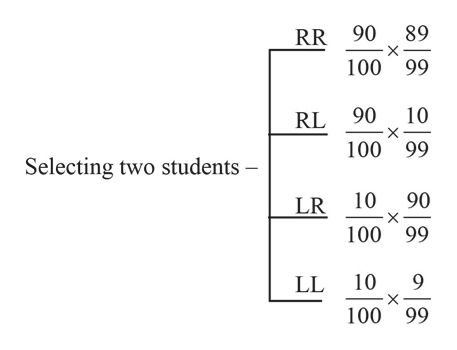# Suppose there are 100 students in your accounting class, 10 of whom are left-handed. Two students are drawn at random. Draw the probability tree and insert the probability for each branch.What is the probability of the following events? Both are right-handed?Both are left-handed?One is right-handed and the other is left-handed?At least one is right-handed?

Question
479 views
1. Suppose there are 100 students in your accounting class, 10 of whom are left-handed.

1. Two students are drawn at random. Draw the probability tree and insert the probability for each branch.

What is the probability of the following events?

1. Both are right-handed?
2. Both are left-handed?
3. One is right-handed and the other is left-handed?
4. At least one is right-handed?
check_circle

Step 1

1)The letter R represents right-handed student and L represents left-handed student. The probability tree and corresponding probabilities is given below:help_outlineImage Transcriptionclose89 RR 90 100 99 RL 90 100 99 10 X Selecting two students - 10 90 LR X 100 99 LL 10 X 100 99 fullscreen
Step 2

Probability of given events:

1)The probability that both student selected are right-handed is calculated as follows:

Step 3

2)The probability that both student selected ar...

### Want to see the full answer?

See Solution

#### Want to see this answer and more?

Solutions are written by subject experts who are available 24/7. Questions are typically answered within 1 hour.*

See Solution
*Response times may vary by subject and question.
Tagged in

### Other